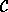## CGAL::Arrangement_with_history_2<Traits,Dcel>

### Definition

An object arr of the class Arrangement_with_history_2<Traits,Dcel> represents the planar subdivision induced by a set of input curves. The arrangement is represented as a doubly-connected edge-list (DCEL). As is the case for the Arrangement_2<Traits,Dcel>, each DCEL vertex is associated with a point and each edge is associated with an x-monotone curve whose interior is disjoint from all other edges and vertices. Each such x-monotone curve is a subcurve of some C- or may represent an overlap among several curves in.

The Arrangement_with_history_2<Traits,Dcel> class-template extends the Arrangement_2 class-template by keeping an additional container of input curves representing, and by maintaining a cross-mapping between these curves and the arrangement edges they induce. This way it is possible to determine the inducing curve(s) of each arrangement edge. This mapping also allows the traversal of input curves, and the traversal of edges induced by each curve.

The Arrangement_with_history_2<Traits,Dcel> template has two parameters:

• The Traits template-parameter should be instantiated with a model of the ArrangementTraits_2 concept. The traits class defines the Curve_2 type, which represents an input curve. It also defines the types of x-monotone curves and two-dimensional points, namely X_monotone_curve_2 and Point_2, respectively, and supports basic geometric predicates on them.
• The Dcel template-parameter should be instantiated with a class that is a model of the ArranagementDcel concept. The value of this parameter is by default Arr_default_dcel<Traits>.

### Inherits From

Arrangement_2<Traits,Dcel>

#include <CGAL/Arrangement_with_history_2.h>

### Types

 typedef Arrangement_with_history_2 Self; a private type used as an abbreviation of the Arrangement_with_history_2 type hereafter.

 Arrangement_with_history_2::Traits_2 the traits class in use. Arrangement_with_history_2::Dcel the DCEL representation of the arrangement.

 typedef typename Traits_2::Point_2 Point_2; the point type, as defined by the traits class. typedef typename Traits_2::X_monotone_curve_2 X_monotone_curve_2; the x-monotone curve type, as defined by the traits class. typedef typename Traits_2::Curve_2 Curve_2; the curve type, as defined by the traits class.

In addition, the nested types Vertex, Halfedge and Face are defined, as well as all handle, iterator and circualtor types, as defined by the Arrangement_2 class-template .

 Arrangement_with_history_2::Curve_handle a handle for an input curve. Arrangement_with_history_2::Curve_iterator a bidirectional iterator over the curves that induce the arrangement. Its value-type is Curve_2. Arrangement_with_history_2::Induced_edge_iterator an iterator over the edges induced by an input curve. Its value type is Halfedge_handle. Arrangement_with_history_2::Originating_curve_iterator an iterator for the curves that originate a given arrangement edge. Its value type is Curve_handle.

### Creation

 Arrangement_with_history_2 arr; constructs an empty arrangement containing one unbounded face, which corresponds to the whole plane. Arrangement_with_history_2 arr ( Self other); copy constructor. Arrangement_with_history_2 arr ( Traits_2 *traits); constructs an empty arrangement that uses the given traits instance for performing the geometric predicates.

### Assignment Methods

 Self& arr = other assignment operator. void arr.assign ( Self other) assigns the contents of another arrangement. void arr.clear () clears the arrangement.

### Access Functions

See the Arrangement_2 referrence pages  for the full list.Accessing the Input Curves:

 Size arr.number_of_curves () returns the number of input curves that induce the arrangement. Curve_iterator arr.curves_begin () returns the begin-iterator of the curves inducing the arrangement. Curve_iterator arr.curves_end () returns the past-the-end iterator of the curves inducing the arrangement. Size arr.number_of_induced_edges ( Curve_handle ch) returns the number of arrangement edges induced by the curve ch. Induced_edge_iterator arr.induced_edges_begin ( Curve_handle ch) returns the begin-iterator of the edges induced by the curve ch. Induced_edge_iterator arr.induced_edges_end ( Curve_handle ch) returns the past-the-end iterator of the edges induced by the curve ch. Size arr.number_of_originating_curves ( Halfedge_handle e) returns the number of input curves that originate the edge e. Originating_curve_iterator arr.originating_curves_begin ( Halfedge_handle e) returns the begin-iterator of the curves originating the edge e. Originating_curve_iterator arr.originating_curves_end ( Halfedge_handle e) returns the past-the-end iterator of the curves originating the edge e.

### Modifiers

See the Arrangement_2 referrence pages  for the full list of functions for modifying arrangement vertices.Modifying Arrangement Edges:

The following functions override their counterparts in the Arrangement_2 class, as they also maintain the cross-relationships between the input curves and the edges they induce.

Halfedge_handle arr.split_edge ( Halfedge_handle e, Point_2 p)
splits the edge e into two edges (more precisely, into two halfedge pairs), at a given split point p. The function returns a handle for the halfedge whose source is the same as e->source() and whose target vertex is the split point.
 Precondition: p lies in the interior of the curve associated with e.

Halfedge_handle arr.merge_edge ( Halfedge_handle e1, Halfedge_handle e2)
merges the edges represented by e1 and e2 into a single edge. The function returns a handle for one of the merged halfedges.
 Precondition: e1 and e2 share a common end-vertex, of degree 2, and the x-monotone curves associated with e1 and e2 are mergeable into a single x-monotone curves.

Face_handle arr.remove_edge ( Halfedge_handle e, bool remove_source = true, bool remove_target = true)
removes the edge e from the arrangement. Since the e may be the only edge incident to its source vertex (or its target vertex), this vertex can be removed as well. The flags remove_source and remove_target indicate whether the endpoints of e should be removed, or whether they should be left as isolated vertices in the arrangement. If the operation causes two faces to merge, the merged face is returned. Otherwise, the face to which the edge was incident is returned.

### See Also

ArrangementDcel
Arr_default_dcel<Traits>
ArrangementTraits_2
Arrangement_2<Traits,Dcel>
insertion functions
removal functions
overlaying arrangements